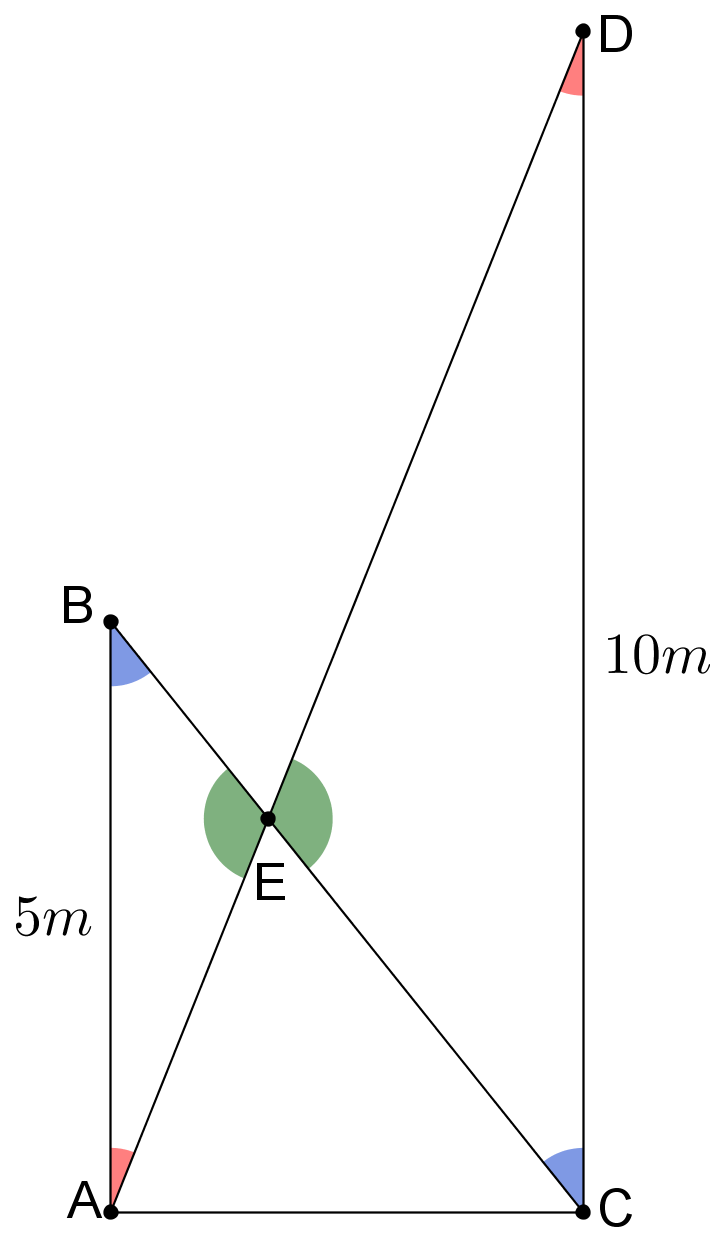#### You may also like### Fitting In

The largest square which fits into a circle is ABCD and EFGH is a square with G and H on the line CD and E and F on the circumference of the circle. Show that AB = 5EF. Similarly the largest equilateral triangle which fits into a circle is LMN and PQR is an equilateral triangle with P and Q on the line LM and R on the circumference of the circle. Show that LM = 3PQ### Look Before You Leap

Can you spot a cunning way to work out the missing length?### Triangle Midpoints

You are only given the three midpoints of the sides of a triangle. How can you construct the original triangle?

##### Age 14 to 16 Challenge Level:

Rhiannon sent in this excellent solution:When the ladders both have their tops $5m$ above the ground, the diagram has a line of symmetry, shown in the first diagram as a dotted line, since the ladders both start and end at the same height.

This means the point of intersection must be halfway between the bottom and the top of each of the ladders, and therefore must be $2.5m$ above the ground.

I expected to need to know how far the bases of the ladders were apart, so not needing to know this surprised me.When the second ladder is $10m$ above the ground, I used a different approach. Since both the walls are perpendicular to the ground, they must be parallel.

This tells me that the angles $\angle BAD$ and $\angle ADC$ are alternate angles, and therefore equal. Likewise, angles $\angle ABC$ and $BCD$ are also alternate and therefore equal.

$\angle BEA$ and $\angle CED$ are opposite, which means that they are equal. You can see these equal angles in the diagram on the right.

This means that the triangles $ABE$ and $DCE$ have the same angles as each other, and are therefore similar.

This means:$$\frac{AE}{ED}=\frac{AB}{DC}=\frac{5m}{10m}=\frac{1}{2}$$
Therefore $AE = \frac{1}{3}AD$.

This means $E$ is $\tfrac{1}{3}$ of the way up from the floor to $D$, so is at a height of $\frac{10m}{3}=3\tfrac{1}{3}m$

If instead $B$ is $a$ metres above the floor, then:$$\frac{AE}{ED}=\frac{AB}{DC}=\frac{a}{10}$$Therefore $AE = \frac{a}{10+a}AD$.

This means $E$ is the same fraction of the $10m$ above the floor, so is $\frac{10a}{10+a}$ metres above the floor.

If instead $D$ is $b$ metres above the floor, then:$$\frac{AE}{ED}=\frac{AB}{DC}=\frac{a}{b}$$Therefore $AE = \frac{a}{a+b}AD$.

This means $E$ is the same fraction of the $b$ metres above the floor, so is $\frac{ab}{a+b}$ metres above the floor.